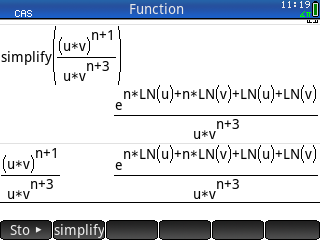Simplifying Rational Expressions - Printable Version +- HP Forums (https://www.hpmuseum.org/forum) +-- Forum: HP Calculators (and very old HP Computers) (/forum-3.html) +--- Forum: HP Prime (/forum-5.html) +--- Thread: Simplifying Rational Expressions (/thread-17226.html) Simplifying Rational Expressions - sascha - 07-08-2021 08:28 AM How can I simplify the expression below with the HP Prime? I like to see u^n over v^2 as the result.RE: Simplifying Rational Expressions - rawi - 07-08-2021 09:18 AM If I make simplify((u^(n+1)*v^(n+1))/(u*v^(n+3))) I get a little bit further to u^(n+1)/(u*v^2), but it is not able to reduce the fraction to u^n/v^2. If I try to simplify the exptression u^(n+1)/(u*v^2) I get what you already got. BTW: If I try expand(((u*v)^(n+1))/(u*v^(n+3))) with the TI89 Titanium I get (u*v)^n/v^(n+2). If I do expand((u^(n+1)*v^(n+1))/(u*v^(n+3))) I get u^n/v^2. So this is not perfect but better than the Prime. Result with TI nspire CX II-T CAS: expand(((u*v)^(n+1))/(u*v^(n+3))) --> (u*v)^n/(u*v^(n+3)) expand((u^(n+1)*v^(n+1))/(u*v^(n+3))) --> u^n/v^2 So it seams that the brackets in the numerator make things more difficult. It's really strange because it is mathematically so simple. Best Raimund RE: Simplifying Rational Expressions - robmio - 07-08-2021 09:38 AM Try to use, in sequence, "texpand" and then "simplify" Greetings, Robmio RE: Simplifying Rational Expressions - sascha - 07-08-2021 09:58 AM (07-08-2021 09:38 AM)robmio Wrote:  Try to use, in sequence, "texpand" and then "simplify" Greetings, Robmio That's what I get: [attachment=9625] RE: Simplifying Rational Expressions - robmio - 07-08-2021 10:26 AM Hi, you don't have to use "expand", but "texpand", followed by "simplify". RE: Simplifying Rational Expressions - Albert Chan - 07-08-2021 11:59 AM CAS> simplify(pow2exp((u*v)^(n+1)/(u*v^(n+3)))) ﻿ ﻿ ﻿ ﻿ ﻿ ﻿ ﻿ ﻿ ﻿ ﻿ ﻿ ﻿ ﻿ ﻿ → u^n/v^2 CAS> simplify(pow2exp(u^(n+1)*v^(n+1)/(u*v^(n+3)))) ﻿ ﻿ ﻿ ﻿ ﻿ ﻿ → u^n/v^2 RE: Simplifying Rational Expressions - sascha - 07-08-2021 12:18 PM (07-08-2021 10:26 AM)robmio Wrote:  Hi, you don't have to use "expand", but "texpand", followed by "simplify". Thanks! Amazing, this works: [attachment=9627] RE: Simplifying Rational Expressions - sascha - 07-08-2021 12:23 PM (07-08-2021 11:59 AM)Albert Chan Wrote:  CAS> simplify(pow2exp((u*v)^(n+1)/(u*v^(n+3)))) ﻿ ﻿ ﻿ ﻿ ﻿ ﻿ ﻿ ﻿ ﻿ ﻿ ﻿ ﻿ ﻿ ﻿ → u^n/v^2 CAS> simplify(pow2exp(u^(n+1)*v^(n+1)/(u*v^(n+3)))) ﻿ ﻿ ﻿ ﻿ ﻿ ﻿ → u^n/v^2 This works great. Thanks! [attachment=9628] RE: Simplifying Rational Expressions - Hlib - 07-09-2021 03:11 PM My afx-2.0 calculator outputs: simplify ((U×V)^(N+1)/((U×V)^(N+3))) result is 1/(U^2×V^2) Is this correct? EDIT My input mistake: (u×v)^(n+1)/(u×v^(n+3)) Yes, Prime wins. RE: Simplifying Rational Expressions - dah145 - 07-09-2021 07:07 PM (07-09-2021 03:11 PM)Hlib Wrote:  My afx-2.0 calculator outputs: simplify ((U×V)^(N+1)/((U×V)^(N+3))) result is 1/(U^2×V^2) Is this correct? The original expression is ((U*V)^(N+1))/(U*(V^(N+3)), my algebra FX 2.0 isn't able to simplify it. RE: Simplifying Rational Expressions - Hlib - 07-09-2021 07:27 PM Yes, I have edit my mistake.